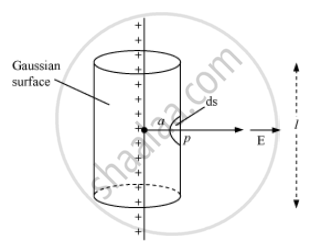Share

# Use Gauss' Law to Derive the Expression for the Electric Field (Vece)Due to a Straight Uniformly Charged Infinite Line of Charge Density λ C/M. - CBSE (Science) Class 12 - Physics

#### Question

Use Gauss' law to derive the expression for the electric field (vecE)due to a straight uniformly charged infinite line of charge density λ C/m.

#### Solution

Field due to an infinitely long straight uniformly charged wireConsider a thin, infinitely long straight line charge of linear charge density λ.

Let P be the point at a distance a from the line. To find the electric field at point P, draw a cylindrical surface of radius ‘a’ and length l.

If E is the magnitude of electric field at point P, then electric flux through the Gaussian surface,

Φ = E × Area of the curved surface of a cylinder of radius r and length l

As electric lines of force are parallel to end faces (circular caps) of the cylinder, there is no component of the field along the normal to the end faces.

Φ = E × 2πal … (i)

According to Gauss's Theorem,

phi = q/epsilon_0

∵ q = lambdal

:. phi = (lambdal)/epsilon_0  ...(ii)

From equations (i) and (ii), we get:

E xx 2pial = (lambdal)/epsilon_0

Is there an error in this question or solution?

#### Video TutorialsVIEW ALL 

Solution Use Gauss' Law to Derive the Expression for the Electric Field (Vece)Due to a Straight Uniformly Charged Infinite Line of Charge Density λ C/M. Concept: Applications of Gauss’s Law.
S